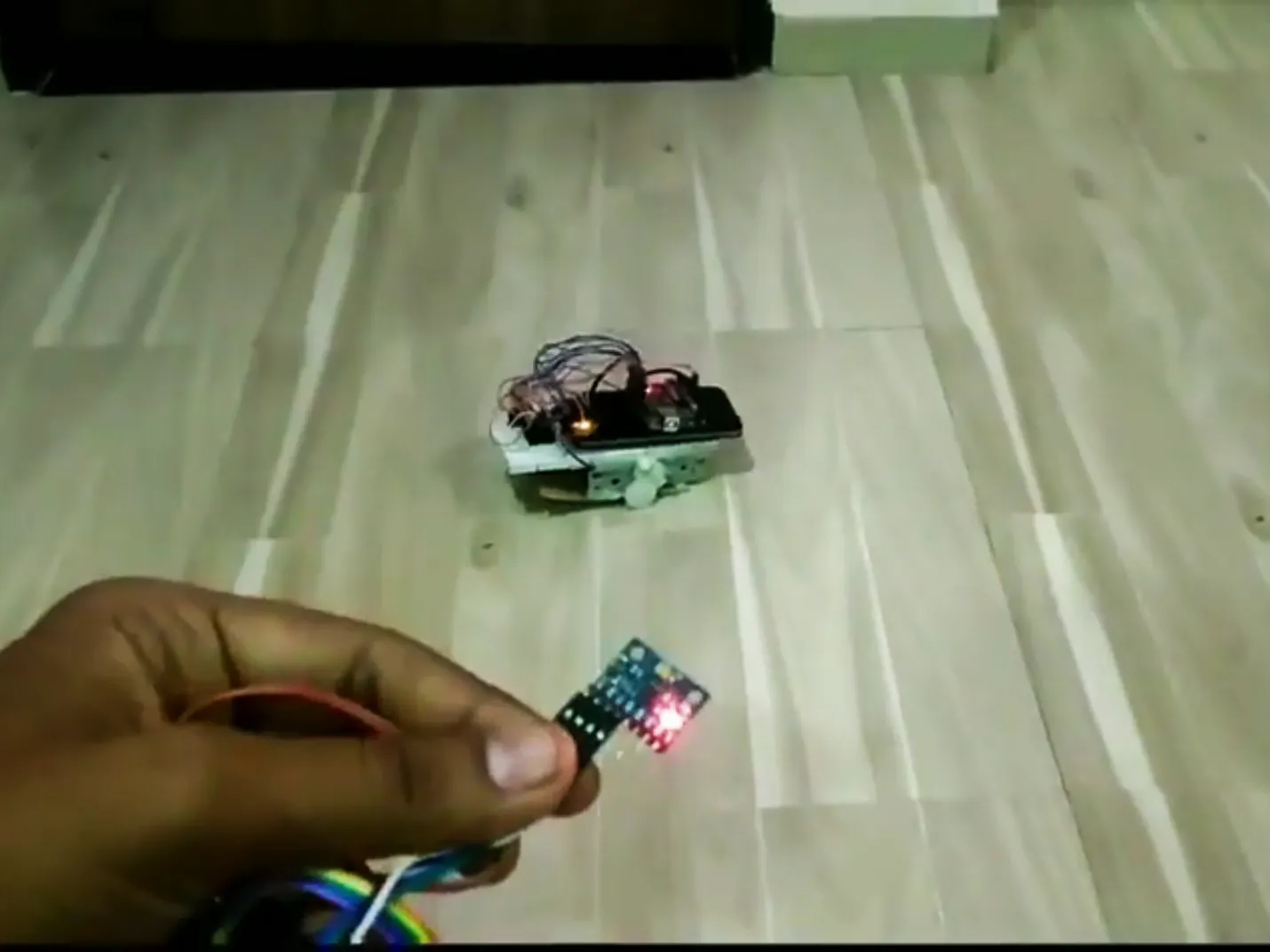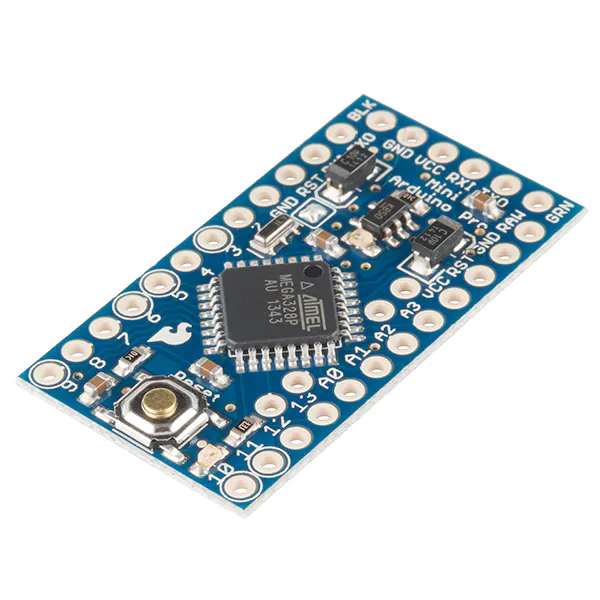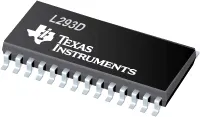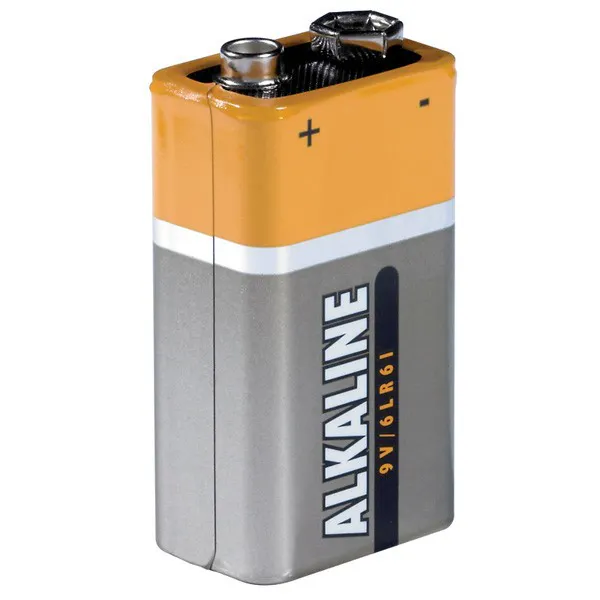Published

# Gesture-Controlled Car

A hand gesture-controlled car made from scrap DVD player. Hand gestures detected by gyro and Arduino-driven motor.

IntermediateShowcase (no instructions)20 hours9,523## Things used in this project

### Hardware componentsArduino UNO & Genuino UNO Used in Receiver side
×1SparkFun Arduino Pro Mini 328 - 5V/16MHz Used in Transmitter side.
×1
 GY-521 MPU-6050 3 Axis Gyroscope + Accelerometer Module For Arduino For "X" & "Y" axis reading measurement.
×1Texas Instruments Dual H-Bridge motor drivers L293D Used for motor speed and direction control
×1
 3.7 V LiPo Battery To power up transmitter
×19V battery (generic)
×1Jumper wires (generic)
×1

### Software apps and online servicesArduino IDE

## Schematics

### GESTURE_Transmitter Schematic

.Fzz file which can open in Fritzing

### GESTURE_Reciever Schematic

.Fzz file which can open in Fritzing
In Schematic i have shown 3.7 volt battery but it is of 5 VDC power bank of 10000 mah.

## Code

### GESTURE_Transmitter.ino

Arduino
This file need to load in Arduino Pro mino(Transmitter).
MPU6050 readings fatched over I2C and used kalman filter before transmitting "X "& "Y" data to Receiving circuit.
For Data transmitting NRF24L01 used.
This cod is originally Kalman library Example code. i just add NRF24L01 library for data transfer to receiver side.
This file required "I2C.ino" file placed in same folder for proper working.
```/*
*NRF module connection
CE    -> 9
CSN   -> 10 (Hardware SPI SS)
MOSI  -> 11 (Hardware SPI MOSI)
MISO  -> 12 (Hardware SPI MISO)
SCK   -> 13 (Hardware SPI SCK)
IRQ   -> No connection in this example
VCC   -> No more than 3.6 volts
GND   -> GND
*/
/*
*NRF module connection
MPU6050 -> Arduino
SDA  -> 4 (Hardware SPI MOSI)
SCL  -> 5 (Hardware SPI MISO)
VCC   -> No more than 3.6 volts
GND   -> GND
*/
//NRF Start
#include <SPI.h> // NRFLite uses Arduino SPI when used with an ATmega328.  This include is not necessary when using an ATtiny84/85.
#include <NRFLite.h>
const static uint8_t RADIO_ID             = 1; // Our radio's id.  Can be any 8-bit number (0-255).
const static uint8_t DESTINATION_RADIO_ID = 0; // Id of the radio we will transmit to.
const static uint8_t PIN_RADIO_CE         = 9;
const static uint8_t PIN_RADIO_CSN        = 10;
uint8_t Counter0; // For sending X value over NRF radio
uint8_t Counter1;};// For sending X value over NRF radio
//NRF End
unsigned long oldTime;
#include <Wire.h>
#include <Kalman.h> // Source: https://github.com/TKJElectronics/KalmanFilter
Kalman kalmanX; // Create the Kalman instances
Kalman kalmanY;
/* IMU Data */
double accX, accY, accZ;
double gyroX, gyroY, gyroZ;
int16_t tempRaw;
double gyroXangle, gyroYangle; // Angle calculate using the gyro only
double compAngleX, compAngleY; // Calculated angle using a complementary filter
double kalAngleX, kalAngleY; // Calculated angle using a Kalman filter
uint32_t timer;
uint8_t i2cData; // Buffer for I2C data
// TODO: Make calibration routine
void setup() {
Serial.begin(115200);
//NRF START
else {
while (1) {} // Wait here forever.}
//NRF END
Wire.begin();
#if ARDUINO >= 157
Wire.setClock(100000UL); // Set I2C frequency to 400kHz
#else
TWBR = ((F_CPU / 100000UL) - 16) / 2; // Set I2C frequency to 400kHz
#endif
i2cData = 7; // Set the sample rate to 1000Hz - 8kHz/(7+1) = 1000Hz
i2cData = 0x00; // Disable FSYNC and set 260 Hz Acc filtering, 256 Hz Gyro filtering, 8 KHz sampling
i2cData = 0x00; // Set Gyro Full Scale Range to 250deg/s
i2cData = 0x00; // Set Accelerometer Full Scale Range to 2g
while (i2cWrite(0x19, i2cData, 4, false)); // Write to all four registers at once
while (i2cWrite(0x6B, 0x01, true)); // PLL with X axis gyroscope reference and disable sleep mode
if (i2cData != 0x68) { // Read "WHO_AM_I" register
while (1);
}
delay(100); // Wait for sensor to stabilize
/* Set kalman and gyro starting angle */
accX = (int16_t)((i2cData << 8) | i2cData);
accY = (int16_t)((i2cData << 8) | i2cData);
accZ = (int16_t)((i2cData << 8) | i2cData);
// Source: http://www.freescale.com/files/sensors/doc/app_note/AN3461.pdf eq. 25 and eq. 26
// atan2 outputs the value of - to  (radians) - see http://en.wikipedia.org/wiki/Atan2
// It is then converted from radians to degrees
#ifdef RESTRICT_PITCH // Eq. 25 and 26
double roll  = atan2(accY, accZ) * RAD_TO_DEG;
double pitch = atan(-accX / sqrt(accY * accY + accZ * accZ)) * RAD_TO_DEG;
#else // Eq. 28 and 29
double roll  = atan(accY / sqrt(accX * accX + accZ * accZ)) * RAD_TO_DEG;
double pitch = atan2(-accX, accZ) * RAD_TO_DEG;
#endif
kalmanX.setAngle(roll); // Set starting angle
kalmanY.setAngle(pitch);
gyroXangle = roll;
gyroYangle = pitch;
compAngleX = roll;
compAngleY = pitch;
timer = micros();
}
void loop() {
/* Update all the values */
accX = (int16_t)((i2cData << 8) | i2cData);
accY = (int16_t)((i2cData << 8) | i2cData);
accZ = (int16_t)((i2cData << 8) | i2cData);
tempRaw = (int16_t)((i2cData << 8) | i2cData);
gyroX = (int16_t)((i2cData << 8) | i2cData);
gyroY = (int16_t)((i2cData << 8) | i2cData);
gyroZ = (int16_t)((i2cData << 8) | i2cData);;
double dt = (double)(micros() - timer) / 1000000; // Calculate delta time
timer = micros();
// Source: http://www.freescale.com/files/sensors/doc/app_note/AN3461.pdf eq. 25 and eq. 26
// atan2 outputs the value of - to  (radians) - see http://en.wikipedia.org/wiki/Atan2
// It is then converted from radians to degrees
#ifdef RESTRICT_PITCH // Eq. 25 and 26
double roll  = atan2(accY, accZ) * RAD_TO_DEG;
double pitch = atan(-accX / sqrt(accY * accY + accZ * accZ)) * RAD_TO_DEG;
#else // Eq. 28 and 29
double roll  = atan(accY / sqrt(accX * accX + accZ * accZ)) * RAD_TO_DEG;
double pitch = atan2(-accX, accZ) * RAD_TO_DEG;
#endif
double gyroXrate = gyroX / 131.0; // Convert to deg/s
double gyroYrate = gyroY / 131.0; // Convert to deg/s
#ifdef RESTRICT_PITCH
// This fixes the transition problem when the accelerometer angle jumps between -180 and 180 degrees
if ((roll < -90 && kalAngleX > 90) || (roll > 90 && kalAngleX < -90)) {
kalmanX.setAngle(roll);
compAngleX = roll;
kalAngleX = roll;
gyroXangle = roll;
} else
kalAngleX = kalmanX.getAngle(roll, gyroXrate, dt); // Calculate the angle using a Kalman filter
if (abs(kalAngleX) > 90)
gyroYrate = -gyroYrate; // Invert rate, so it fits the restriced accelerometer reading
kalAngleY = kalmanY.getAngle(pitch, gyroYrate, dt);
#else
// This fixes the transition problem when the accelerometer angle jumps between -180 and 180 degrees
if ((pitch < -90 && kalAngleY > 90) || (pitch > 90 && kalAngleY < -90)) {
kalmanY.setAngle(pitch);
compAngleY = pitch;
kalAngleY = pitch;
gyroYangle = pitch;
} else
kalAngleY = kalmanY.getAngle(pitch, gyroYrate, dt); // Calculate the angle using a Kalman filter
if (abs(kalAngleY) > 90)
gyroXrate = -gyroXrate; // Invert rate, so it fits the restriced accelerometer reading
kalAngleX = kalmanX.getAngle(roll, gyroXrate, dt); // Calculate the angle using a Kalman filter
#endif
gyroXangle += gyroXrate * dt; // Calculate gyro angle without any filter
gyroYangle += gyroYrate * dt;
//gyroXangle += kalmanX.getRate() * dt; // Calculate gyro angle using the unbiased rate
//gyroYangle += kalmanY.getRate() * dt;
compAngleX = 0.93 * (compAngleX + gyroXrate * dt) + 0.07 * roll; // Calculate the angle using a Complimentary filter
compAngleY = 0.93 * (compAngleY + gyroYrate * dt) + 0.07 * pitch;
// Reset the gyro angle when it has drifted too much
if (gyroXangle < -180 || gyroXangle > 180)
gyroXangle = kalAngleX;
if (gyroYangle < -180 || gyroYangle > 180)
gyroYangle = kalAngleY;
/* Print Data */
int x = kalAngleX* -1 ;
int y = kalAngleY * -1 ;
Serial.print(x); Serial.print("\t");
Serial.print("\t");
Serial.print(y); Serial.print("\t");
Serial.print("\r\n");
delay(2);
//NRF START
if(oldTime-millis() > 500){
//      Serial.println("...Success");
}
else {//Serial.println("...Failed");
}
oldTime = millis();
}
}
```

### Gesture control transmitter I2C.ino

Arduino
Need to place this file in same folder where GESTURE_Transmitter.ino file is saved.
```/* Copyright (C) 2012 Kristian Lauszus, TKJ Electronics. All rights reserved.

This software may be distributed and modified under the terms of the GNU
Foundation and appearing in the file GPL2.TXT included in the packaging of
this file. Please note that GPL2 Section 2[b] requires that all works based
on this software must also be made publicly available under the terms of
the GPL2 ("Copyleft").

Contact information
-------------------

Kristian Lauszus, TKJ Electronics
Web      :  http://www.tkjelectronics.com
e-mail   :  kristianl@tkjelectronics.com
*/

const uint8_t IMUAddress = 0x68; // AD0 is logic low on the PCB
const uint16_t I2C_TIMEOUT = 1000; // Used to check for errors in I2C communication

uint8_t i2cWrite(uint8_t registerAddress, uint8_t data, bool sendStop) {
return i2cWrite(registerAddress, &data, 1, sendStop); // Returns 0 on success
}

uint8_t i2cWrite(uint8_t registerAddress, uint8_t *data, uint8_t length, bool sendStop) {
Wire.write(data, length);
uint8_t rcode = Wire.endTransmission(sendStop); // Returns 0 on success
if (rcode) {
Serial.print(F("i2cWrite failed: "));
Serial.println(rcode);
}
return rcode; // See: http://arduino.cc/en/Reference/WireEndTransmission
}

uint32_t timeOutTimer;
uint8_t rcode = Wire.endTransmission(false); // Don't release the bus
if (rcode) {
Serial.println(rcode);
return rcode; // See: http://arduino.cc/en/Reference/WireEndTransmission
}
Wire.requestFrom(IMUAddress, nbytes, (uint8_t)true); // Send a repeated start and then release the bus after reading
for (uint8_t i = 0; i < nbytes; i++) {
if (Wire.available())
else {
timeOutTimer = micros();
while (((micros() - timeOutTimer) < I2C_TIMEOUT) && !Wire.available());
if (Wire.available())
else {
return 5; // This error value is not already taken by endTransmission
}
}
}
return 0; // Success
}
```

### GESTURE_RECIEVER.ino

Arduino
This file need to be load in Receiver Arduino which is installed on Car.
In this Arduino Received two reading from NRF24L01 which actually send by transmitter part. one reading is X axis value and second is Y axis reading.
according to X & Y reading Motor direction and speed are controlled for to achieve desired motion of Car.
```/*
*NRF module connection
CE    -> 9
CSN   -> 10 (Hardware SPI SS)
MOSI  -> 11 (Hardware SPI MOSI)
MISO  -> 12 (Hardware SPI MISO)
SCK   -> 13 (Hardware SPI SCK)
IRQ   -> No connection in this example
VCC   -> No more than 3.6 volts
GND   -> GND

*H Bridge L293D Connectioon

H-Bridge -> Arduino
1   -> 5  (RHS motor PWM)
2   -> 2  (RHS motor Direction a)
7   -> 3  (RHS motor Direction B)
9   -> 6  (LHS motor PWM)
15  -> 4  (LHS motor Direction a)
10  -> 7  (LHS motor Direction a)
*/
#include <SPI.h> // NRFLite uses Arduino SPI when used with an ATmega328.  This include is not necessary when using an ATtiny84/85.

int mot1en = 5 ;   // RHS motor PWM
int mot2en = 6 ;   // LHS motor PWM
int mot1dira = 2;  // RHS motor direction a
int mot1dirb = 3;  // RHS motor direction b
int mot2dira = 4;  // LHS motor direction a
int mot2dirb = 7;  // LHS motor direction b
int NRF_connt = 8; // NRF interface working status led
bool for_rev_en_bit,lft_rt_en_bit,for_bit,rev_bit,lft_bit,rt_bit; // motor control bit will derived from gyro signal
double for_rev,lft_rt; // Actual angle value of "X" and "Y" axis recieved from transmitter
byte count;
int datapac,led_state;
const static uint8_t RADIO_ID      = 0;   // Our radio's id.  The transmitter will send to this id.
const static uint8_t PIN_RADIO_CE  = 9;   // CE pin of NRF
const static uint8_t PIN_RADIO_CSN = 10;  // CSN pin of NRF
struct RadioPacket1 {  // Data structure for NRF recieved data storage. it shoud match with Transmitter NRF Data Structure.
uint8_t GYROX;
uint8_t GYROY;
};
void setup()
{
delay(500); // Give the serial monitor a little time to get ready so it shows all serial output.
Serial.begin(115200);
//Motor setup
pinMode(mot1en,OUTPUT);
pinMode(mot2en,OUTPUT);
pinMode(mot1dira,OUTPUT);
pinMode(mot1dirb,OUTPUT);
pinMode(mot2dira,OUTPUT);
pinMode(mot2dirb,OUTPUT);
pinMode(mot2en,OUTPUT);
pinMode(NRF_connt,OUTPUT);
//Motor setup closed
}

else {
while (1) {} // Wait here forever.
}
}
void loop()
{
uint8_t packetSize = _radio.hasData(); // hasData returns 0 if there is data packet.
if(for_rev>169 &  for_rev <= 255){ for_rev = map(for_rev,169,255,-86,0);}
if(lft_rt>169 &  lft_rt <= 255){ lft_rt = map(lft_rt,169,255,-86,0);}
}
if(count <= 200){
datapac = datapac + packetSize;
count ++;
}
if(count >= 201){
if(datapac == 0){for_rev = 0; lft_rt = 0;count = 0;led_state= LOW;Serial.println("NRF fail");}
else {datapac =0; count=0;Serial.println("NRF working");led_state = HIGH;}
}
if (for_rev > -6 & for_rev < 6 ){
for_rev_en_bit = LOW;
}
else {
for_rev_en_bit = HIGH;
if(for_rev >6){for_bit = HIGH; }else {for_bit = LOW;}
if(for_rev <-6){rev_bit = HIGH;} else {rev_bit = LOW;}
}
if (lft_rt > -6 & lft_rt < 6){  //no speed variation moment
lft_rt_en_bit = LOW;
}
else{
lft_rt_en_bit = HIGH;
if(lft_rt >6)lft_bit = HIGH; else lft_bit = LOW;
if(lft_rt <-6)rt_bit = HIGH; else rt_bit = LOW;
}
if (for_rev_en_bit == HIGH & for_bit == HIGH)forward();
else if (for_rev_en_bit == HIGH & rev_bit == HIGH)revarse();
//else if (for_rev_en_bit == LOW & lft_rt_en_bit == HIGH & lft_bit == HIGH)leftonly();
//else if (for_rev_en_bit == LOW & lft_rt_en_bit == HIGH & rt_bit == HIGH)rightonly();
else {stopped();}
digitalWrite(NRF_connt,led_state);
}
void forward(){
digitalWrite(mot1dira,HIGH);
digitalWrite(mot1dirb,LOW);
digitalWrite(mot2dira,HIGH);
digitalWrite(mot2dirb,LOW);
//Serial.println("forward on");
if (lft_rt_en_bit == LOW){
int x = map(for_rev,6,20,80,255);
x = constrain(x,80,255);
analogWrite(mot1en,x);
analogWrite(mot2en,x);
Serial.print("lft rt bit,");Serial.print(lft_rt_en_bit);Serial.print(",");Serial.print(x);
}
if (lft_rt_en_bit == HIGH & lft_bit == HIGH){
int x = map(for_rev,6,20,80,255);
int y = map(lft_rt,6,50,80,255);
x = constrain(x,80,255);
y = constrain(y,50,255);
analogWrite(mot1en,x-y);
analogWrite(mot2en,x);
}
if (lft_rt_en_bit == HIGH & rt_bit == HIGH){
int x = map(for_rev,6,20,80,255);
int y = map(lft_rt,-6,-50,80,255);
x = constrain(x,80,255);
y = constrain(y,50,255);
analogWrite(mot1en,x);
analogWrite(mot2en,x-y);
}
}
void revarse(){
digitalWrite(mot1dira,LOW);
digitalWrite(mot1dirb,HIGH);
digitalWrite(mot2dira,LOW);
digitalWrite(mot2dirb,HIGH);
//Serial.println("reverse on");
if (lft_rt_en_bit == LOW){
int x = map(for_rev,-6,-20,80,255);
x = constrain(x,80,255);
analogWrite(mot1en,x);
analogWrite(mot2en,x);
Serial.print("lft rt bit,");Serial.print(lft_rt_en_bit);Serial.print(",");Serial.print(x);
}
if (lft_rt_en_bit == HIGH & lft_bit == HIGH){
int x = map(for_rev,-6,-20,80,255);
int y = map(lft_rt,6,50,80,255);
x = constrain(x,80,255);
y = constrain(y,80,255);
analogWrite(mot1en,x-y);
analogWrite(mot2en,x);
}
if (lft_rt_en_bit == HIGH & rt_bit == HIGH){
int x = map(for_rev,-6,-20,80,255);
int y = map(lft_rt,-6,-50,80,255);
x = constrain(x,80,255);
y = constrain(y,80,255);
analogWrite(mot1en,x);
analogWrite(mot2en,x-y);
}
}
void leftonly(){
digitalWrite(mot1dira,LOW);
digitalWrite(mot1dirb,HIGH);
digitalWrite(mot2dira,HIGH);
digitalWrite(mot2dirb,LOW);
//Serial.println("Only right on");
analogWrite(mot1en,180);
analogWrite(mot2en,180);}
void rightonly(){
digitalWrite(mot1dira,HIGH);
digitalWrite(mot1dirb,LOW);
digitalWrite(mot2dira,LOW);
digitalWrite(mot2dirb,HIGH);
//Serial.println("Only left on");
analogWrite(mot1en,180);
analogWrite(mot2en,180);}
void stopped(){
analogWrite(mot1en,0);
analogWrite(mot2en,0);
digitalWrite(mot1dira,LOW);
digitalWrite(mot1dirb,LOW);
digitalWrite(mot2dira,LOW);
digitalWrite(mot2dirb,LOW);
//Serial.println("Stopped");
}
```